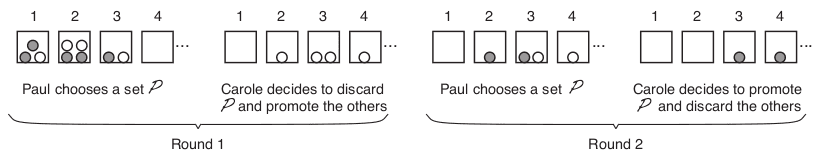### B - Boxes and Stones

##### Languages: C, C++, Java, Pascal, ... (details)

Paul and Carole like to play a game with $S$ stones and $B$ boxes numbered from $1$ to $B$. Before beginning the game they arbitrarily distribute the $S$ stones among the boxes from $1$ to $B - 1$, leaving box $B$ empty. The game then proceeds by rounds. At each round, first Paul chooses a subset $\mathcal{P}$ of the stones that are in the boxes; he may choose as many stones as he wants from as many boxes as he wants, or he may choose no stones at all, in which case $\mathcal{P}$ is empty. Then, Carole decides what to do next: she can either promote the subset $\mathcal{P}$ and discard the remaining stones (that is, those stones not chosen by Paul in the first step); or she may discard the subset $\mathcal{P}$ and promote the remaining stones. To promote a given subset means to take each stone in this subset and move it to the box with the next number in sequence, so that if there was a stone in this subset inside box $b$, it is moved to box $b + 1$. To discard a given subset means to remove every stone in this subset from its corresponding box, so that those stones are not used in the game for the remaining rounds. The figure below shows an example of the first two rounds of a game.Paul and Carole play until at least one stone reaches box number $B$, in which case Paul wins the game, or until there are no more stones left in the boxes, in which case Carole wins the game. Paul is a very rational player, but Carole is a worthy rival because she is not only extremely good at this game, but also quite lucky. We would like to know who is the best player, but before that we must first understand how the outcome of a game depends on the initial distribution of the stones. In particular, we would like to know in how many ways the $S$ stones can initially be distributed among the first $B - 1$ boxes so that Carole can be certain that she can win the game if she plays optimally, even if Paul never makes a mistake.

#### Input

Each test case is described using one line. The line contains two integers $S$ $(1 \leq S \leq 200)$ and $B$ $(2 \leq B \leq 100)$, representing respectively the number of stones and the number of boxes in the game.

#### Output

For each test case output a line with an integer representing the number of ways in which the $S$ stones may be distributed among the first $B - 1$ boxes so that Carole is certain that she can win the game. Because this number can be very large, you are required to output the remainder of dividing it by $10^9 + 7$.

Input
2 3 8 4 42 42
Output
2 0 498467348